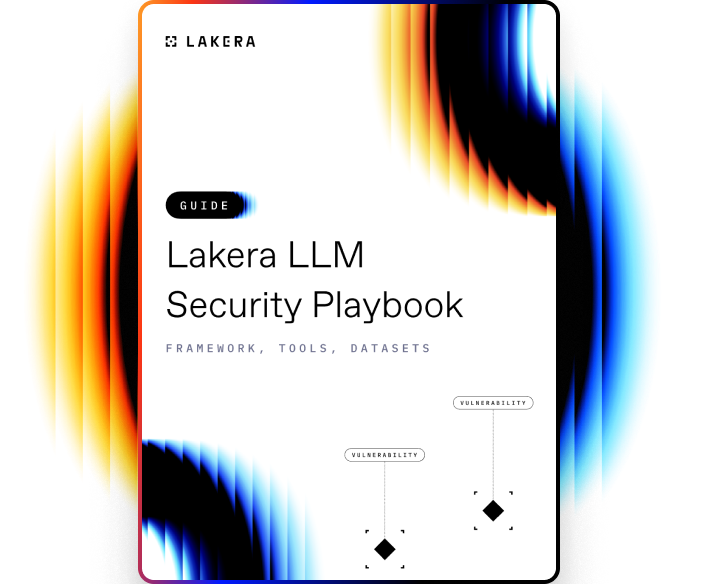VOCABULARY

# Calibration Curve

A calibration curve in machine learning refers to a graphical representation that demonstrates how the predicted probabilities of a classification model align with the actual outcomes. Ideally, in a well-calibrated model, if a prediction says there's a 70% chance of a particular event happening, then that event should indeed occur approximately 70% of the time.

Calibration curves help in assessing the reliability of the predicted probabilities.

## How Calibration Curve works

To understand and plot a calibration curve:

1. Binning Predicted Probabilities: Start by segmenting or "binning" the predicted probabilities into discrete intervals (e.g., 0-0.1, 0.1-0.2, etc.).
2. Compute Actual Frequencies: For each bin, calculate the actual frequency or proportion of positive examples. For instance, in the 0.7-0.8 bin, if there are 100 predictions and 75 of them are true positives, then the actual positive frequency is 0.75.
3. Plotting: On a graph, the x-axis represents the mean predicted probability for each bin, and the y-axis represents the actual observed frequency of positives. For a perfectly calibrated model, the calibration curve will be a 45-degree diagonal line, often referred to as the "line of perfect calibration."
4. Assess Model Calibration: If the curve lies above the line of perfect calibration, the model is under-confident in its predictions. If it's below, the model is over-confident.
5. Calibration Techniques: If a model is found to be miscalibrated, there are techniques like Platt Scaling, Isotonic Regression, and Beta Calibration that can be applied to adjust the predicted probabilities to be more aligned with the true outcomes.

The calibration curve thus provides a visualization of how trustworthy a model's probability estimates are, enabling practitioners to make more informed decisions based on those probabilities.Learn how to protect against the most common LLM vulnerabilities

Download this guide to delve into the most common LLM security risks and ways to mitigate them.

Related termsActivate
untouchable mode.